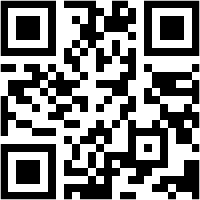Need Help? Chat with us

# CountBlank Function in Excel

## How to use Countblank function in Excel?

This tutorial will give you knowledge regarding the CountBlank function in Excel. “CountBlank function counts the number of cells that are empty in the spreadsheet or in a particular table.” Count blank function does not count text, numbers, space or errors. They count only the empty cells in the spreadsheet. The output of this function is that it represents a number regarding empty cells in the worksheet.

## Special Offer for First Time Users

If you are the first time user and want to learn Microsoft office skills, then you can avail this special offer discount on your email.Syntax or the Formula of CountBlank

The formula for using Countblank function is

=COUNTBLANK(range)

Here only one parameter is required to fulfill the Count blank function in Excel. Here the range stands for the cell or range of cells you want to count empty cells for.

? How does Countblank Function work in Excel?

We are not very much interested in counting blank cells in Excel. However if at times we need to calculate how many empty cells are there in Excel worksheet we will use the Formula and count it. Counting empty cells becomes difficult when we have a large amount of data.

Let’s look into the example: As we can see in the video that how many empty cells are in the range (B4: D6) we get the answer as 2.

? Points to Remember:

While we count the no of blank cells in the spreadsheet we must keep the following points in mind as to what will be counted in Count blank function and what will not be counted.

1. Cells that contain text, numbers, errors, space, etc. are not counted.

2. Cells that contain zero are also not counted.

3. If the cell contains an empty text string or a formula that returns empty text string will be counted in the CountBlank function in Excel.

4. The Count Blank function is not satisfactory in these two circumstances when cells of interest are in the closed workbook and when they do not form a contiguous range. So in such a situation, we use SUM (IF) function.

Properly Delete the Cells so that it is not Counted

After selecting a cell we need to erase the contents in the cell, this can be done with the help pf backspace key or the delete key. “Always use the Delete key while deleting values in the cell.” This is because even space is considered as a value in the cell.

We might not be able to see the space in the cell and count it as empty. However, when we use the formula we get a different result. So to avoid such confusion we must always use the delete key while deleting values from the cell.

CountBlank and Counta function in Excel

Counta function counts the number of cells that are not empty whereas Countblank function in excel will count the number of cells that are empty. Both the functions are an individual function of Count.

Where Do you Find CountBlank function in Excel?

Countblank function is found in the formula bar when you start typing in the formula bar.

You can also find this function Insert function.

Countblank function is found in the Formula Tab > Statistical function > Countblank.

An alternate function of CountBlank:

The alternative function of count blank is Count IF. Count IF counts the number of a cell in the range that does not contain any value and return numbers in return. Cells that contain text, numbers, errors, etc. are not counted. The same as Count Blank function in Excel.

The Countblank function is an in-built function in Excel. It is categorized in the Statistical function. It is a worksheet function and can also be entered in the formula bar of the worksheet. It was first introduced in the year 2000. The above description might have given you enough information regarding the use of Count blank function in Excel.

Hope you like our tutorial for more cool and amazing trick of excel Like our Excel Superstar Facebook Page and subscribe to our Excel Superstar YouTube channel. Excel Superstar is the leading online training company, which provides Online Excel Course in Hindi. Connect with us and become an Excel Superstar.